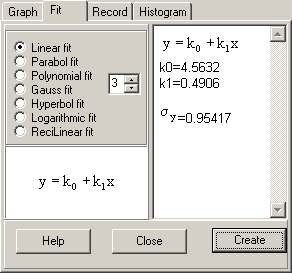If you have an idea, that your data fits with a special datatype, you can select the most important here and evaluate the corresponding parameters.=the square of the correlation coefficient .

In the example above a linear fit is selected.
So the datas are near the line
y=4.3963+0.51279*x